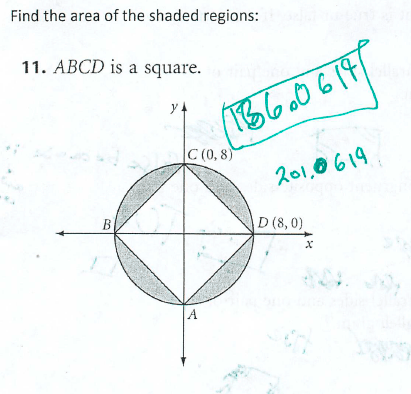Categories

# Area of a Circle, Minus a SquareDo you see the mistake? How would you help this student?

## 2 replies on “Area of a Circle, Minus a Square”

Isaaksays:

In haste, presumably, the student miscalculated the area of the square to be 64 as if the side length were 8 whereas the area of the square shown is twice that of a square of side length 8. The formula for the area of a square is so easy to use, the student jumped in to start using it without thinking enough about whether the number 8 that was so handy for the area of the circle was also right value for the square’s area formula..

One might help by ask them to draw four squares each of area 16 at the same scale, but on a different set of axes and aligned with the axes.. Ask whether their result is consistent or inconsistent with the square shown here, and why their areas compare the way they do. Ask whether the area of a square changes as it rotates.

If that doesn’t trigger the alarm bells, ask the student to visualize cutting the square along the axes into triangles, and moving the two lower triangles (for instance), diagonally across the origin. The resulting shape makes it obvious there is more area than a square of side length 8.

Or one could go ahead and ask them how they might be able to compute the side length CD.

To get at the overall problem-solving skill of asking if your answer makes sense, the first question might be, is more or less than half of the area in each sector shaded? Qualitatively they might guess it is less than half. To which one could ask, so does your answer make sense?

To target the specific misconception of miscalculating the square, have the student draw the Square with diagonal CD on the diagram. They should be able to respond that the area of that square is 64sq un, and see that the unshaded triangle is half of it, with an area of 32sq un. They can see the large unshaded square is composed of 4 such triangles, and must have an area of 4*32 = 128sq un. Even though no units are specified in the problem, I would recommend un^2 be added to their response.